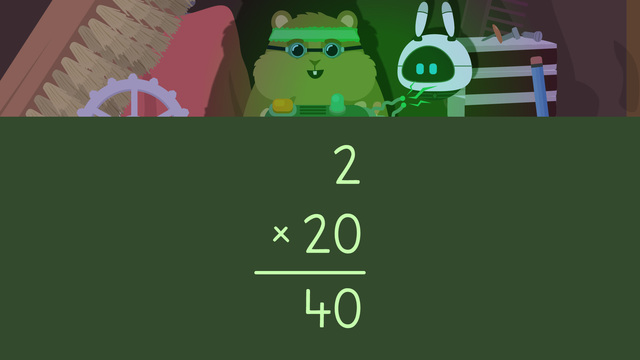# Multiplying TensRate this video

Ø 5.0 / 2 ratings

The authorTeam Digital
Multiplying Tens
CCSS.MATH.CONTENT.3.NBT.A.3

## Information about the videoMultiplying Tens

### In This Video on Multiplying by 10s

Mr. Squeaks is low on items in his workshop, so has created a multiplier machine that multiplies items by multiples of ten! In the end, Mr. Squeaks decides to use it to multiply some cheese, and accidentally sits down on the machine, which is facing Imani! Watch this multiplying by 10s video to learn how to multiply by tens!

### Multiplying by Whole 10s Example

Let’s take a look at this example from the above math video teaching multiplying by 10s, two times twenty.When multiplying by 10s, we write a zero in the ones place as a placeholder, since any number multiplied by zero will always be zero.Next, we move to the next place value, the tens place, and we multiply the two by the two above.Two times two is four, so we write this in the tens place next to the zero. Now we have finished solving! Two times twenty gives a product of forty, which is the solution to the problem.### Multiplying by 10s Review

To multiply by tens, follow these steps;

• Set up the problem, using standard algorithm.
• Write a zero in the ones place as a placeholder.
• Multiply the tens place digit by the single digit and write the product next to the zero.Below, you will find a multiplying by 10s worksheet for further practice.

### TranscriptMultiplying Tens

Mr. Squeaks has built a handheld clone machine, which works by multiplying single digit objects by multiples of tens! Mr. Squeaks wants to calculate the final product first so that he doesn't clone too many objects. Mr. Squeaks needs help multiplying tens. A multiple of ten is a number with a zero in the ones place. Ten, twenty, thirty, and so on are all multiples of ten. To multiply a single digit number by a multiple of ten, first, set up the problem, aligning the place values. Next, write a zero in the ones place. A zero is added here because any number multiplied by a multiple of ten will always have a zero in the ones place! Finally, multiply the tens place digit by the single digit. Four times five gives a product of twenty, which means four times fifty is two hundred! Mr. Squeaks only has two metal rods left and wants to clone them twenty times. Set up the problem, writing two times twenty like this. Next, write a zero in the ones place. Now look at the two in the tens place and multiply it by two. Two times two is four, so write it in the tens place. The product of two times twenty is forty! Mr. Squeaks is happy with forty metal rods, so he uses his machine! "This sure is hungry work, but I only have three slices of cheese left!" Let's help multiply his cheese slices by forty, so he has plenty to eat. Write three times forty, aligning the place values. What is the next step? Next, write a zero in the ones place. What should we do next? Look at the four in the tens place and multiply it by three. What is three times four? Three times four is twelve. We write twelve in the hundreds and tens place. Three times forty gives our solution one hundred twenty. One hundred twenty slices of cheese should last Mr. Squeaks a while. Before we join Mr. Squeaks for snack time, let's review! Remember, when multiplying by multiples of ten, first, set up the problem, aligning the place values. Next, write a zero in the ones place. Finally, multiply the tens place digit by the single digit to get the product! "This cheese looks delicious!" Wait, that's not a chair, Mr. Squeaks! "Woah, I definitely don't need that many Imani's!"